×

Search anything:

# Different ways to select random element from list in Python

#### Software Engineering PythonFollowing are the different ways of selecting a random element from a list:

• random.randrange()
• random.random()
• random.choice()
• random.randint()

# 1. random.randrange() :

using this method we can return a random element from the given list.This method generates random value in the specified range.so for lists we need to specify the range as " random.randrange(len(listname)) ".And we will pass the value returned by the above method into our list to genereate the random elementfrom the list.
So basically we are generating a random index value usinh the above method and passing that random index value into our list will generate a random element from the list.

### Complexity

• Worst case time complexity: `Θ(<1>)`
• Average case time complexity: `Θ(<1>)`
• Best case time complexity: `Θ(<1>)`
• Space complexity: `Θ(<1>)`

### Implementations

``````import random
list1 = [10,20,30,70,90,60]
print("the given list is :",list1)
random_index = random.randrange(len(list1))
print("random selected element is",list1[random_index])
``````

### OUTPUT

Each time you will run the code you will get a different output.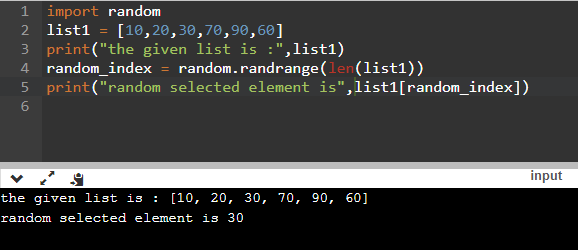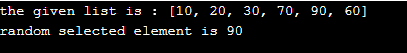### Applications

Random number generators have applications in gambling, statistical sampling, computer simulation, cryptography, completely randomized design, and other areas where producing an unpredictable result is desirable. Generating a random number has always been an important application and having many uses in daily life.Python number method randrange() returns a randomly selected element from range(start, stop, step)

# 2. random.random():

using this method we can return a random element form the list given.

The above method return float value in between the range 0 and 1.so we will generate a random index value for list by multiplying the value returned by the method with the length of string and then converting them into integer in order to get the index value.

### Complexity

• Worst case time complexity: `Θ(<1>)`
• Average case time complexity: `Θ(<1>)`
• Best case time complexity: `Θ(<1>)`
• Space complexity: `Θ(<1>)`

### Implementations

``````import random

list2 = [10, 40, 50, 20,90, 70]
print ("Original list is : " ,list2)
random_index = int(random.random() * len(list2))
print ("Random selected number is : " ,list2[random_index] )
``````

### OUTPUT

Each time you will run the code you will get a different output.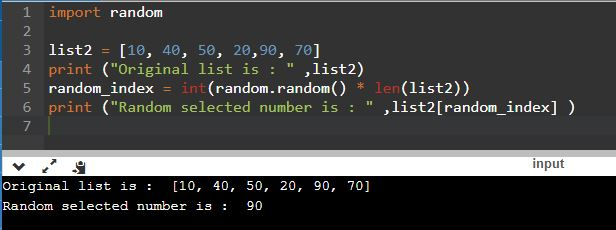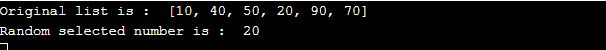### Applications

random() is the most basic function of the random module.
Almost all functions of the random module depend on the basic function random()

# 3. random.choice() :

using this method we can return a random element from the given list.
This method directly generates and returns the value of random element from the given list that is being passed in the method as

``````random.choice(list_name)
``````

### Complexity

• Worst case time complexity: `Θ(<1>)`
• Average case time complexity: `Θ(<1>)`
• Best case time complexity: `Θ(<1>)`
• Space complexity: `Θ(<1>)`

### Implementations

``````import random
list3 = [10,20,30,70,90,60]
print("the given list is :",list3)
random_element = random.choice(list3)
print("random selected element is",random_element)
``````

### OUTPUT

Each time you will run the code you will get a different output.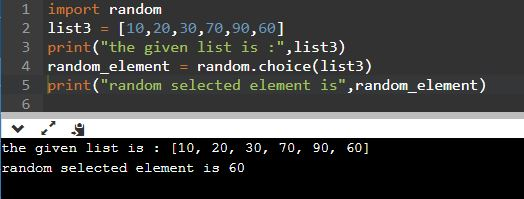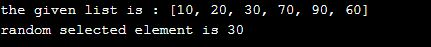### Applications

The choice() method returns a randomly selected element from the specified sequence. The sequence can be a string, a range, a list, a tuple or any other kind of sequence.

# 4. random.randint():

using this method we can return a random element form the list given.
The above method generates a random integer between the specified range 0 and last index of list as "random.randint(0,len(list)-1)". so we will generate a random index value for list and passing that randomly genrated index will genrate the random element from list.

### Complexity

• Worst case time complexity: `Θ(<1>)`
• Average case time complexity: `Θ(<1>)`
• Best case time complexity: `Θ(<1>)`
• Space complexity: `Θ(<1>)`

### Implementations

``````import random

list4 = [10, 40, 50, 20,90, 70]
print ("Original list is : " ,list4)
random_index = random.randint(0,len(list4)-1)
print ("Random selected number is : " ,list4[random_index] )
``````

### OUTPUT

Each time you will run the code you will get a different output.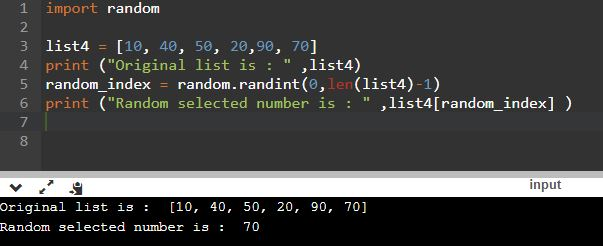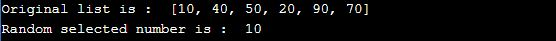### Applications

The randint() function can be used to simulate a lucky draw situation.